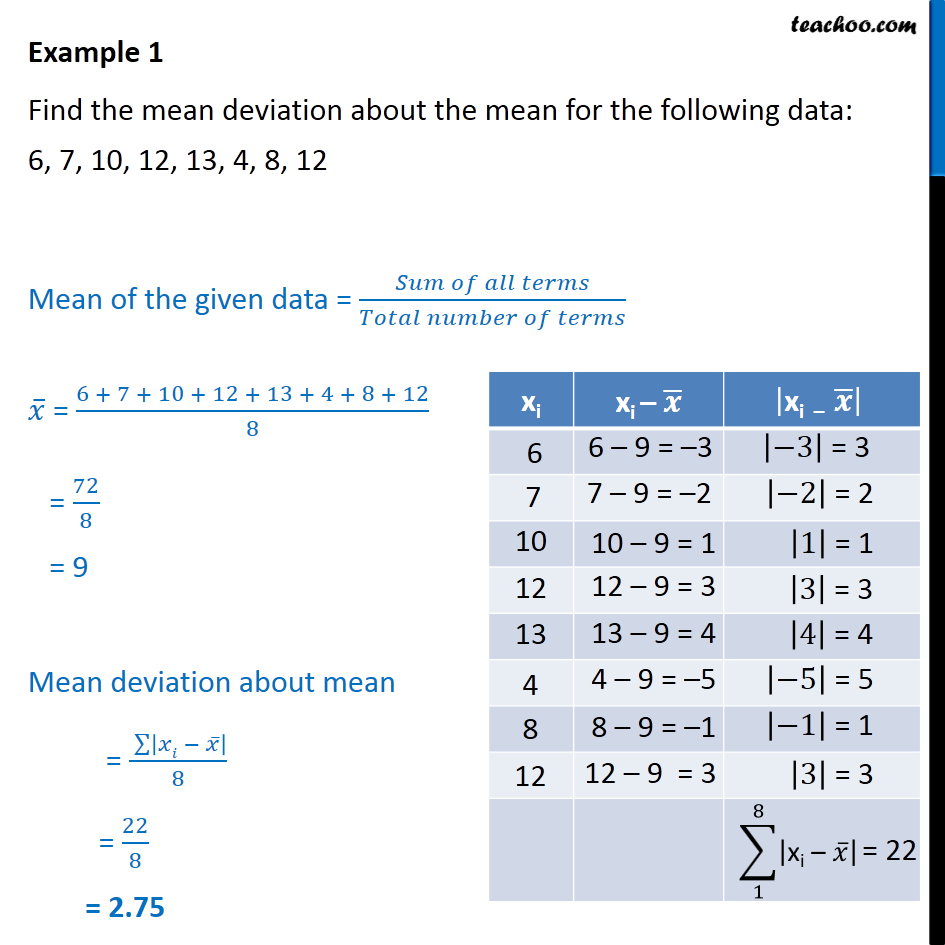Mean deviation about mean - Ungrouped

Chapter 15 Class 11 Statistics
Concept wiseIntroducing your new favourite teacher - Teachoo Black, at only ₹83 per month

### Transcript

Example 1 Find the mean deviation about the mean for the following data: 6, 7, 10, 12, 13, 4, 8, 12 Mean of the given data = = 6 + 7 + 10 + 12 + 13 + 4 + 8 + 12 8 = 72 8 = 9 Mean deviation about mean = 8 = 22 8 = 2.75